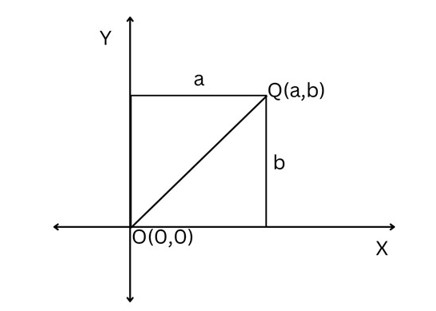# Swift Program to Calculate Distance Between Two Points

This tutorial will discuss how to write swift program to calculate distance between two points.

Suppose we have two points and a line connect these points together. So the length of the line is known as the distance between two points in a plane. This formula is used to find the distance between two points in XYplane.## Formula

Following is the formula −

Distance = sqrt((a2-a1) + (b2-b1))


Below is a demonstration of the same −

Input

Suppose our given input is −

Point 1: (1.0,2.0)
Point 2: (3.0,4.0)


Output

The desired output would be -

Distance between point 1 and point 2: 1.4142135623730951


We can calculate the distance between two points with using in-built library functions. Here we use sqrt() and pow() functions. sqrt() function is used to find the square root of the number whereas pow() function is used to find the power of the given number.

## Algorithm

Following is the algorithm −

Step 1- Create a function with return value.

Step 2- Calculate distance between two points using the following formula −

let res = sqrt(pow((a2-a1), 2) + pow((b2-b1), 2))


Step 3- Calling the function and pass two points in the function as a parameter.

Step 4- Print the output.

## Example

The following program shows how to calculate distance between two points.

import Foundation
import Glibc

// Creating a function to calculate distance between two points.
func Distance(a1: Double, a2: Double, b1: Double, b2: Double) ->Double{
let res = sqrt(pow((a2-a1), 2) + pow((b2-b1), 2))
return res
}

// Points
var m1 = 4.0
var m2 = 6.0
var n1 = 5.0
var n2 = 8.0
print("Point 1: (\(m1),\(m2))")
print("Point 2: (\(n1),\(n2))")
print("Distance between point 1 and point 2:", Distance(a1:m1, a2:m2, b1:n1, b2:n2))


## Output

Point 1: (4.0,6.0)
Point 2: (5.0,8.0)
Distance between point 1 and point 2: 3.605551275463989


Here, in the above program we create a function which return the distance between two points using the following formula −

let res = sqrt(pow((a2-a1), 2) + pow((b2-b1), 2))


Here, we use sqrt() function to find the square root and pow() function is used to find power.